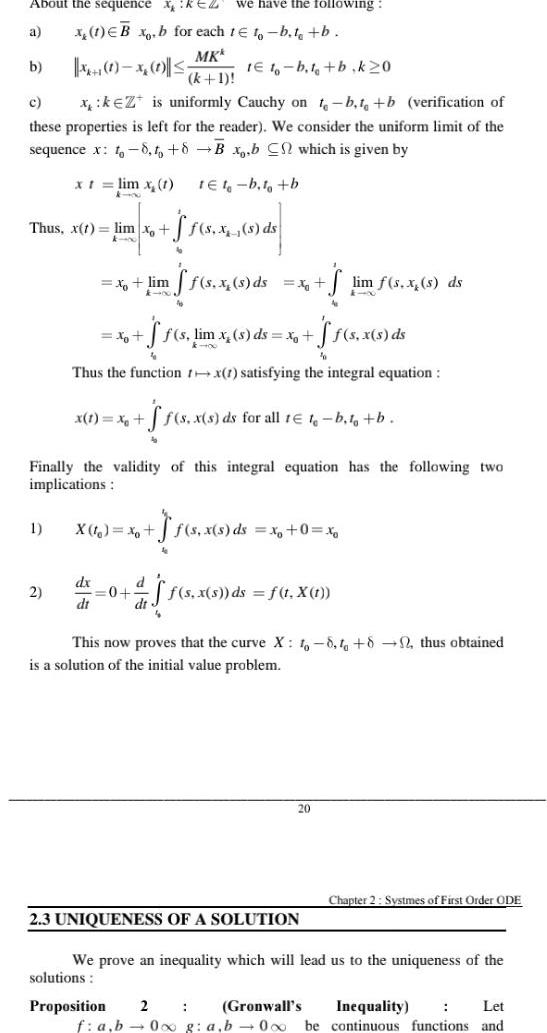Question:

# About the sequence XKEZ we have the following x 1 EBx b for

Last updated: 9/18/2023About the sequence XKEZ we have the following x 1 EBx b for each 1 to b t b MKk a X 1 1 x 1 k 1 1E10 b t b k 20 c x k Z is uniformly Cauchy on t b t b verification of these properties is left for the reader We consider the uniform limit of the sequence x to 8 to 8 B x b C which is given by tet b t b b Thus x t lim xo kin x t lim x 1 4 00 1 2 x lim ff s x s ds 76 x A f s x s ds S s lim x s ds x ff s x s ds Thus the function rx 1 satisfying the integral equation x 1 x S f s x s ds for all te t b to b Finally the validity of this integral equation has the following two implications 4 x X t xo f s x s ds x 0 xo 50 4 S A dx d 0 af f s x s ds f t X 1 di dt Proposition 2 This now proves that the curve X 1 8 1 82 thus obtained is a solution of the initial value problem 20 2 3 UNIQUENESS OF A SOLUTION lim f s x s ds We prove an inequality which will lead us to the uniqueness of the solutions Gronwall s f a b 0x g a b 0 o be Chapter 2 Systmes of First Order ODE Let Inequality continuous functions and